Machine Learning Knowledge Center

# Cross Validation

Cross-validation is one of the methods used to improve the generalization performance.

## Purpose of cross-validation

The purpose of cross-validation is to prevent overfitting and to improve generalization performance.

## Types of cross-validation

There are various methods of cross-validation, but here we will discuss the commonly used k-split cross-validation and stratified k-split cross-validation.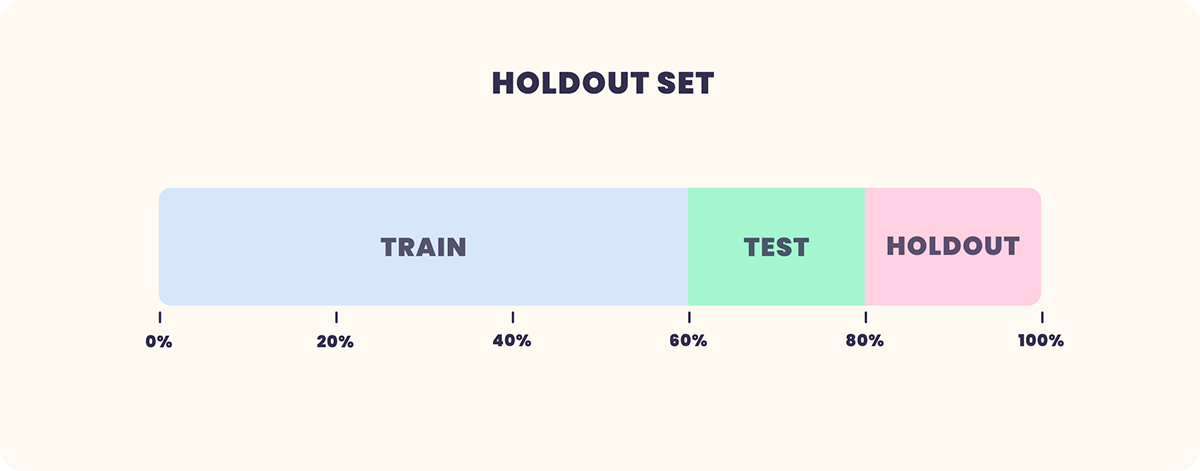### K-Fold Cross Validation: k-Fold Cross Validation

This is a method to train a model by dividing the data into K pieces and using one of them as test data and the remaining K-1 pieces as training data.
In this section, we will use an example of dividing the data into 5 parts.
As shown in the figure below, we divide the data into 5 parts.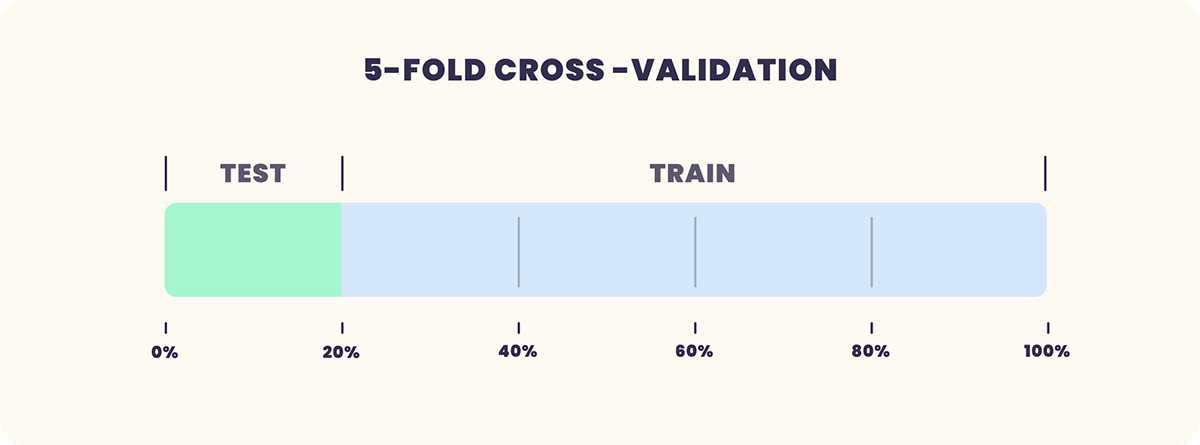Now that we have divided the data into 5 parts, we will train 4 parts for training and 1 part for testing.
This is not the end of training, but we will continue training by shifting the test data position one by one, and finally create 5 models.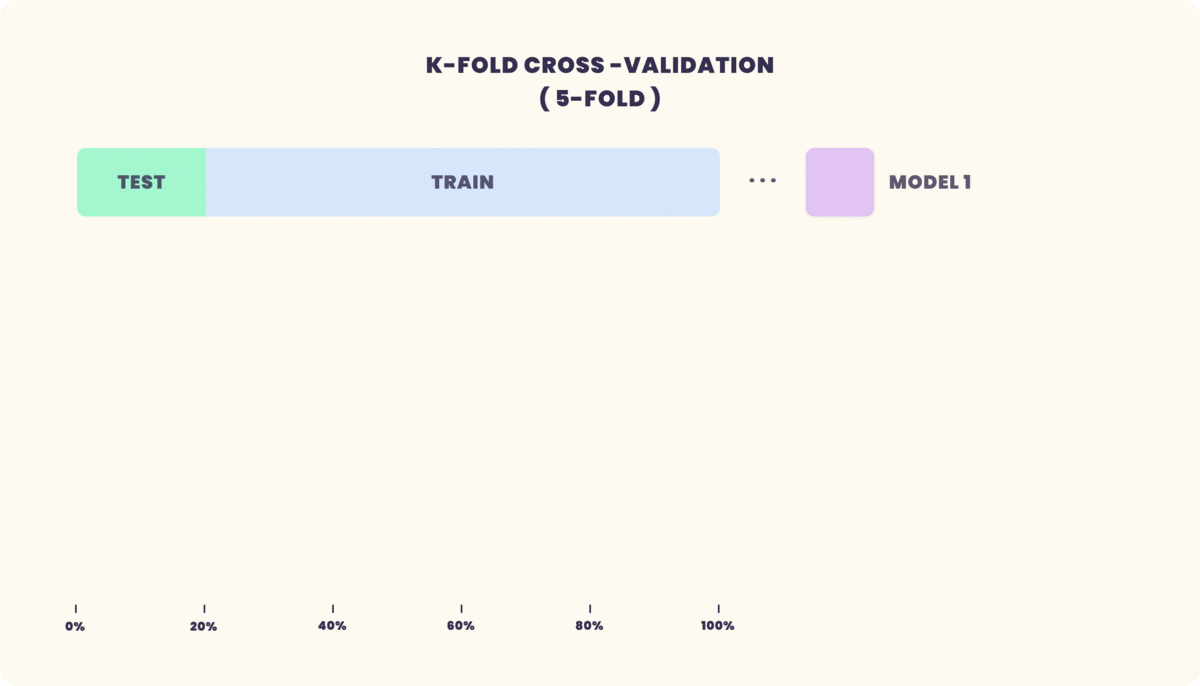Evaluate the accuracy of the resulting five models, average them, and finally fuse them into a single model.
However, since the division is random, data bias is not taken into account, and if there is bias in the number of data in a class in a classification problem, the model performance cannot be evaluated correctly.
Therefore, when there is a bias in the number of data per class included in the data, Stratified k-Fold Cross Validation is used.

### Stratified k-Fold Cross Validation: Stratified k-Fold Cross Validation

The k-fold cross validation simply splits the data, but in this case, the splitting is done in such a way that the ratio of the classes is the same within the split.
The following figure shows the image of data partitioning in stratified k-split cross-validation.
Assuming that we have a data set with different ratios of male and female, if we split the data using this method, the ratios of male and female in each split data range will be the same.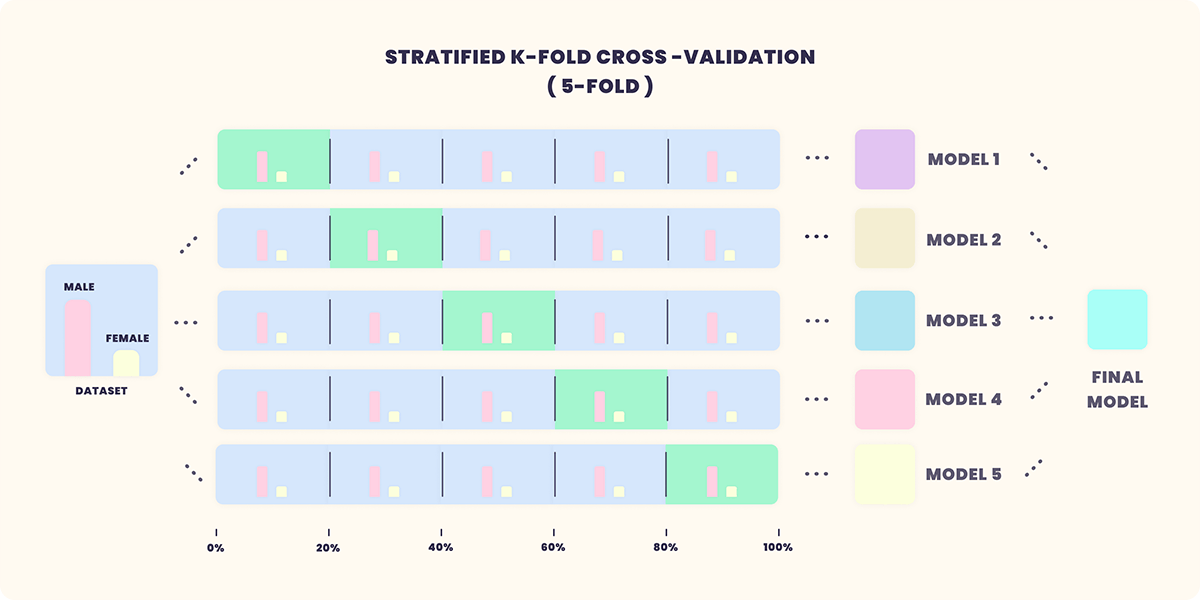It is recommended to use stratified k-split cross-validation instead of k-split cross-validation when creating a model with class biased data.

## Cross-validation in VARISTA

VARISTA also supports cross-validation, and you can check the results after you have finished creating your model.
The following figure shows a detailed screenshot of a model trained using k-partition cross-validation with the data divided into three parts.
The average of the scores in the three models is displayed in the upper right corner.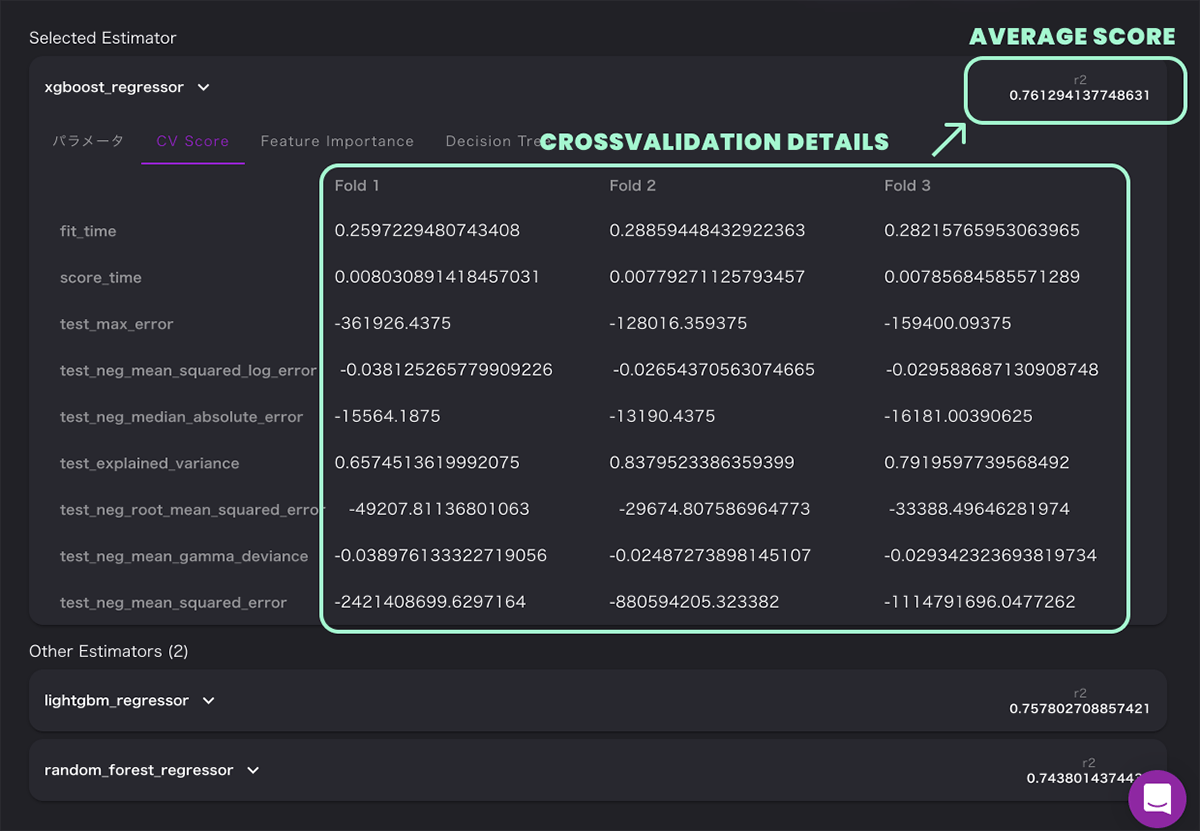VARISTAは機械学習モデルの開発、管理をノーコードで効率的に行うことができる新しいプラットフォームです。
データをお持ちでしたらすぐに始められますので、是非ともお試しください。

or

Stay in Touch